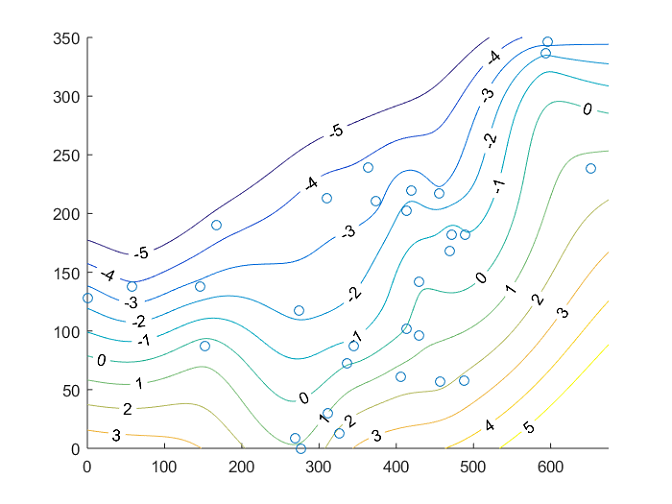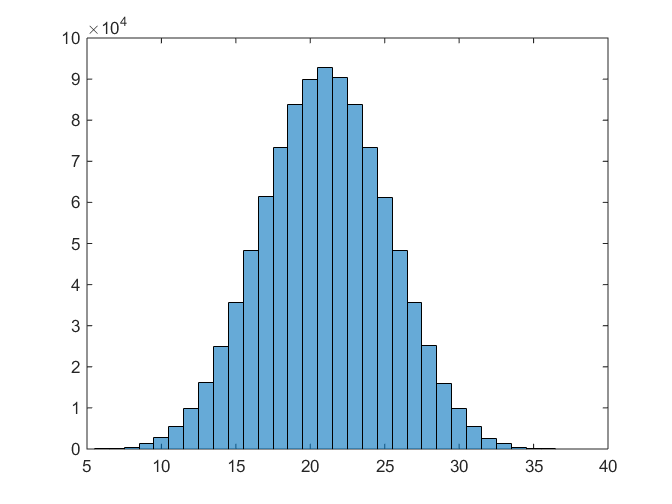# Sample exam problems

Click here for data and code files necessary to complete the practice problems.

1. The provided text file (`readings.txt`) contains a timestamp broken up into year, month, day, hour, minute, second, and timezone components, as well as a reading from a sensor. Write a script that reads the data from the file using the `textscan` function.

###### The script must:
• Convert the timestamps into single numeric serial date numbers stored in a variable named `dates`
• Ignore the timezone component of the timestamp by not reading it into the workspace
• Place numeric values for the readings in a single array of type `double` with a variable name of `readings`
2. ###### The provided data file (`viewdata.mat`) contains a 19-by-3 matrix `viewdata` with the following columns:
1. Video Length - Length in minutes for the video
2. Views - Number of times the video has been viewed
3. Minutes Watched - Total amount of time viewers spent watching the video
###### Write a MATLAB script to analyze the data to determine the effect of video length on viewer retention with the following steps:
• Load the saved viewership data into the MATLAB workspace.
• Create the column vector `viewPct` containing the percentage viewed for each variable according to the formula: Percentage viewed = (Minutes watched / Views) / (Video length).
• Create vectors containing the percentage viewed for short (Video length < 1.5), medium (1.5 <= Video length <= 2.25), and long (Video length > 2.25) videos).
• Calculate the average of the values contained in the vectors from the previous step, and store the results in the variables `shortPct`, `medPct`, and `longPct` respectively.
3. ###### The provided data file (`TData.mat`) contains temperature data reported by weather stations instance in time. The contents of the data file are:
1. The x-coordinates of the weather station locations (in km) stored in the column vector `x`
2. The y-coordinates of the weather station locations (in km) stored in the column vector `y`
3. The temperature data corresponding to the station locations (in degrees C) stored in the column vector `T`

Write a MATLAB script to load the data from the file and produce a contour plot similar to the one below with the temperature stations. Use the `griddata` function with `'v4'` as the interpolation method to estimate the temperatures for the x-y spatial grid points.###### The plot must contain:
• contours spanning -5 degrees C to 5 degrees C in 1 degree increments
• a spatial extent of the contour plot corresponding to 0 < x < 675, 0 < y < 350; with a grid resolution (grid square size) of 1km-by-1km.
• labels for the contours with the `clabel function`
• markers for the weather station locations
4. ###### Create an anonymous function `f` which accepts a (possibly vector valued) numeric input and returns a (possibly vector valued) numeric output according to the mathematical formula f(x) = x^2 - sin(x). Use this function along with the `fminsearch` function to find the local minimum value near the initial value near `x0 = 0.5.` Store the local minimizing value and the corresponding function value in the variables `xmin` and `ymin` respectively.5. ###### A function called `viewImage` accepts an image and a variable number of parameter name/value pairs as shown in the function heading:

`function viewImage (I, varargin)`

###### Write the validation code in the body of the function to produce an error message explaining the violation if any of the following conditions on the input arguments are not met:
• If variable input options exist, they must exist in pairs
• The names portion of the variable input must occur before the corresponding value. Names must be strings of the value `'zoom'`, `'rotate'`, or `'tilt'`
• The value portion for a variable input must be numeric

Do not write any implementation beyond the code required to perform the validation.

6. ###### The provided script `(diceSimulation.m)` runs a simulation of rolling six, 6-sided dice and calculating the sum. The simulation is repeated 1,000,000 times to create a histogram of the probability distribution as shown below.The code produces the correct result, but can be improved to run faster. Rewrite the script such that the simulation produces the same results with 1,000,000 trials, a histogram of the results is created, and a faster execution time.

The figure illustrates on result from running the script. Solutions should have a similar distribution.

7. ###### The provided script `(LoadData.m)` loads data from an impact simulation, and uses the `plotyy` function to plot position on the left y-axis and velocity on the right y-axis. Using the outputs from the `plotyy` function, modify the figure to make it look as shown in the figure below.###### The figure must contain:
• an x-axis with a minimum value of 0 and a maximum value of 4
• a plot of the position vector as a solid blue line
• a left y-axis colored in blue with a minimum value of -5 and a maximum value of 5
• a plot of the velocity vector as a dashed black line
• a right y-axis colored in black with a minimum value of -0.5 and a maximum value of +0.5
• a right y-axis colored in black with a minimum value of -0.5 and a maximum value of +0.5
• a title reading "Impact Data" as shown in the figure
• a legend in the top center of the axes with the correct label for each plot
8. ###### The provided graphical application (see screenshot below) plots a sine wave based on the provided amplitude and frequency using the equation `y = amplitude*sin(2*pi*frequency*x)` on the interval defined by `[0 2*pi].`###### Write the callback functions for the slider controls to update the plot. The callbacks must:
• Update the plot with the new amplitude or frequency
• Update the display of the amplitude and frequency values (tag names `edtAmplitude` and `edtFrequency` respectively.

Additionally, display a plot with the default values for amplitude and frequency upon starting the application.